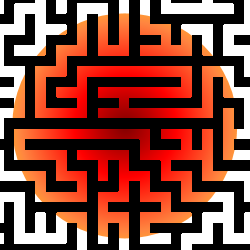Games
Problems
Go Pro!

# An Equation is not a Process

Lesson Plans > Mathematics

## An Equation is not a ProcessSometimes when I'm teaching my math students I'll see them do some astonishing things with equations - things that make my hair (what's left of it) curl, and my blood curdle. Suppose a student wants to multiply 7 by 3, and then add 5. I might see them do something like this:

7 x 3 = 21

Now that is just fine. But then I see them doing this, simply adding to the existing line:

7 x 3 = 21 + 5 = 26

You see what happened there, right? They are treating the equals sign not as an indicator that two sides of the equation have the same value, but as an indicator of a process undertaken. To the student, this looks and feels right. They would read this aloud as "Seven times three equals twenty-one. Then add five and you get twenty-six."

But what they don't realize is that they've actually written 7 x 3 = 21 + 5, or 21 = 26.

This notion that an equals sign represents a process undertaken to obtain an answer is often emphasized in subtle ways. We got my six year old son a cheap calculator to play with. Almost immediately he started referring to the equals sign as the "give me the answer button." He is regarding the equality symbol as a means to an end.

This "equals as process" notion crops up in algebra when most of our introductory equations are things like 2x = 10; x + 4 = 12; and 2x - 3 = 11. What all of these equations have in common is that the variables are all on the left, and a single value us on the right. These equations lend themselves to the notion that the equals sign represents a process undertaken to obtain an answer. It is not until a bit later in most algebra curricula that we start introducing equations which clearly don't have "the answer" on the right: 2x = 3x - 10 or 20 = 4x + 4.

Students end up with a skewed understanding of equations; they tend to regard the two sides of any equation as having different meanings. The left-hand side of an equation is the "question" side of the equation, and the right-hand side is the "answer" side. We should be looking for ways to combat this mentality, and encourage students to think of an equation as a statement rather than a process.

The curriculum my wife is using to teach our son attempts in subtle ways to show that the two sides of an equation are equal not just in value, but also in meaning. One of the simplest ways to do that is to reverse the order of an equation from time to time. Occasionally the book will replace an example like 5 + 5 = 10 with the equivalent example 10 = 5 + 5 (that's just the reflexive property of equality). This may help students break out of the mindset that an equation is a process rather than a statement.

When teaching algebra, we should also do the same. Don't start by exclusively doing problems like 3x + 1 = 10; regularly swap it up and do 10 = 3x + 1. The problem is no easier of harder when written that way, but it may help some students break out of the "equation is process" mentatity.

I try to do the same with word problems. My tendency is to write things like "the sum of two consecutive integers is 45." By I should regularly write problems like "45 is the sum of two consecutive integers."

And if you ever see students doing this:

7 x 3 = 21 + 5 = 26

Tell them it's illegal, immoral, and socially unacceptable, and if they persist in doing it, you'll imprison them in math prison and have them beaten with a mobius strip.

Lesson by Mr. Twitchell# Understanding Coronavirus Spread

A Question and Answer session with Professor Puzzler about the math behind infection spread.

# Blogs on This SiteReviews and book lists - books we love!The site administrator fields questions from visitors.Like us on Facebook to get updates about new resources